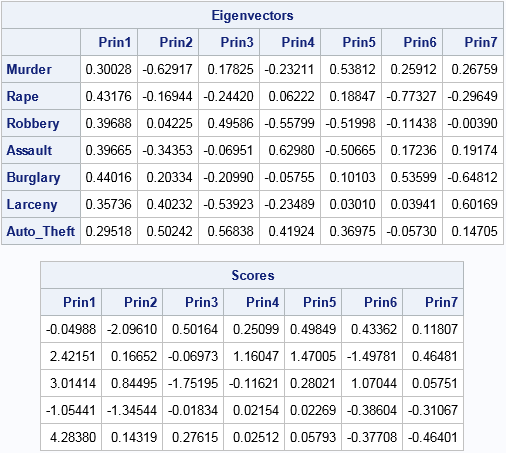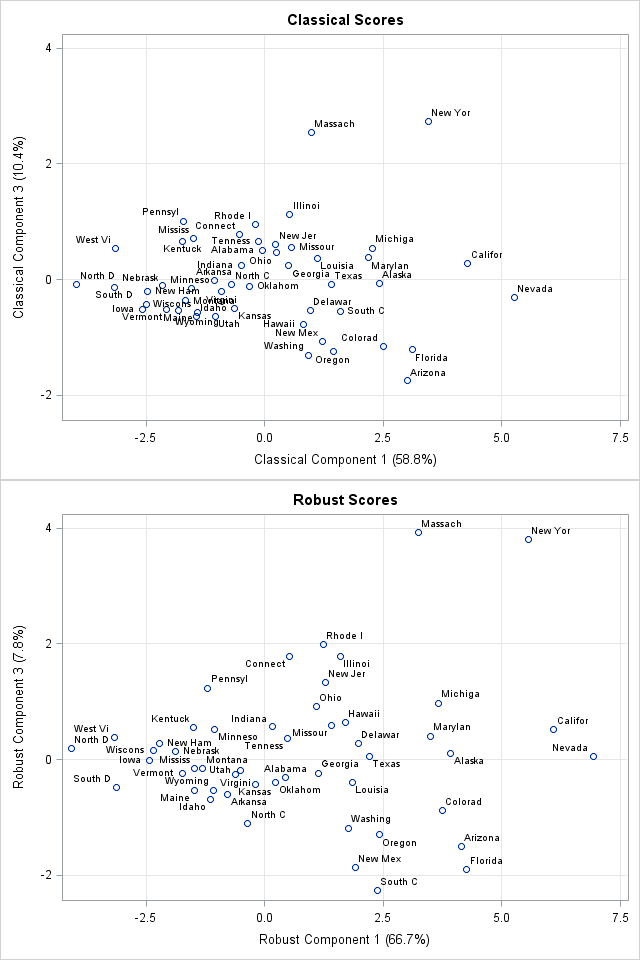Recently, I was asked whether SAS can perform a principal component analysis (PCA) that is robust to the presence of outliers in the data. A PCA requires a data matrix, an estimate for the center of the data, and an estimate for the variance/covariance of the variables. Classically, these estimates are the mean and the Pearson covariance matrix, respectively, but if you replace the classical estimates by robust estimates, you can obtain a robust PCA.

This article shows how to implement a classical (non-robust) PCA by using the SAS/IML language and how to modify that classical analysis to create a robust PCA.

### A classical principal component analysis in SAS

Let's use PROC PRINTCOMP perform a simple PCA. The PROC PRINCOMP results will be the basis of comparison when we implement the PCA in PROC IML. The following example is taken from

 ```proc princomp data=Crime /* add COV option for covariance analysis */ outstat=PCAStats_CORR out=Components_CORR plots=score(ncomp=4); var Murder Rape Robbery Assault Burglary Larceny Auto_Theft; id State; ods select Eigenvectors ScreePlot '2 by 1' '4 by 3'; run;   proc print data=Components_CORR(obs=5); var Prin:; run;```

To save space, the output from PROC PRINCOMP is not shown, but it includes a table of the eigenvalues and principal component vectors (eigenvectors) of the correlation matrix, as well as a plot of the scores of the observations, which are the projection of the observations onto the principal components. The procedure writes two data sets: the eigenvalues and principal components are contained in the PCAStats_CORR data set and the scores are contained in the Components_CORR data set.

### A classical principal component analysis in SAS/IML

Assume that the data consists of n observations and p variables and assume all values are nonmissing. If you are comfortable with multivariate analysis, a principal component analysis is straightforward: the principal components are the eigenvectors of a covariance or correlation matrix, and the scores are the projection of the centered data onto the eigenvector basis. However, if it has been a few years since you studied PCA, Abdi and Williams (2010) provides a nice overview of the mathematics. The following matrix computations implement the classical PCA in the SAS/IML language:

```proc iml; reset EIGEN93; /* use "9.3" algorithm, not vendor BLAS (SAS 9.4m3) */ varNames = {"Murder" "Rape" "Robbery" "Assault" "Burglary" "Larceny" "Auto_Theft"}; use Crime; read all var varNames into X; /* X = data matrix (assume nonmissing) */ close;   S = cov(X); /* estimate of covariance matrix */ R = cov2corr(S); /* = corr(X) */ call eigen(D, V, R); /* D=eigenvalues; columns of V are PCs */ scale = T( sqrt(vecdiag(S)) ); /* = std(X) */ B = (X - mean(X)) / scale; /* center and scale data about the mean */ scores = B * V; /* project standardized data onto the PCs */   print V[r=varNames c=("Prin1":"Prin7") L="Eigenvectors"]; print (scores[1:5,])[c=("Prin1":"Prin7") format=best9. L="Scores"];```The principal components (eigenvectors) and scores for these data are identical to the same quantities that were produced by PROC PRINCOMP. In the preceding program I could have directly computed R = corr(X) and scale = std(X), but I generated those quantities from the covariance matrix because that is the approach used in the next section, which computes a robust PCA.

If you want to compute the PCA from the covariance matrix, you would use S in the EIGEN call, and define B = X - mean(X) as the centered (but not scaled) data matrix.

### A robust principal component analysis

This section is based on a similar robust PCA computation in Wicklin (2010). The main idea behind a robust PCA is that if there are outliers in the data, the covariance matrix will be unduly influenced by those observations. Therefore you should use a robust estimate of the covariance matrix for the eigenvector computation. Also, the arithmetic mean is unduly influenced by the outliers, so you should center the data by using a robust estimate of center before you form the scores.

SAS/IML software provides

 ```/* get robust estimates of location and covariance */ N = nrow(X); p = ncol(X); /* number of obs and variables */ optn = j(8,1,.); /* default options for MCD */ optn= floor(0.75*N); /* override default: use 75% of data for robust estimates */ call MCD(rc,est,dist,optn,x); /* compute robust estimates */ RobCov = est[3:2+p, ]; /* robust estimate of covariance */ RobLoc = est[1, ]; /* robust estimate of location */   /* use robust estimates to perform PCA */ RobCorr = cov2corr(RobCov); /* robust correlation */ call eigen(D, V, RobCorr); /* D=eigenvalues; columns of V are PCs */ RobScale = T( sqrt(vecdiag(RobCov)) ); /* robust estimates of scale */ B = (x-RobLoc) / RobScale; /* center and scale data */ scores = B * V; /* project standardized data onto the PCs */```

Notice that the SAS/IML code for the robust PCA is very similar to the classical PCA. The only difference is that the robust estimates of covariance and location (from the MCD call) are used instead of the classical estimates.

If you want to compute the robust PCA from the covariance matrix, you would use RobCov in the EIGEN call, and define B = X - RobLoc as the centered (but not scaled) data matrix.

### Comparing the classical and robust PCAYou can create a score plot to compare the classical scores to the robust scores. The Getting Started example in the PROC PRINCOMP documentation shows the classical scores for the first three components. The documentation suggests that Nevada, Massachusetts, and New York could be outliers for the crime data.

You can write the robust scores to a SAS data set and used the SGPLOT procedure to plot the scores of the classical and robust PCA on the same scale. The first and third component scores are shown to the right, with abbreviated state names serving as labels for the markers. (Click to enlarge.) You can see that the robust first-component scores for California and Nevada are separated from the other states, which makes them outliers in that dimension. (The first principal component measures overall crime rate.) The robust third-component scores for New York and Massachusetts are also well-separated and are outliers for the third component. (The third principal component appears to contrast murder with rape and auto theft with other theft.)

Because the crime data does not have huge outliers, the robust PCA is a perturbation of the classical PCA, which makes it possible to compare the analyses. For data that have extreme outliers, the robust analysis might not resemble its classical counterpart.

If you run an analysis like this on your own data, remember that eigenvectors are not unique and so there is no guarantee that the eigenvectors for the robust correlation matrix will be geometrically aligned with the eigenvectors from the classical correlation matrix. For these data, I multiplied the second and fourth robust components by -1 because that seems to make the score plots easier to compare.

### Summary

In summary, you can implement a robust principal component analysis by using robust estimates for the correlation (or covariance) matrix and for the "center" of the data. The MCD subroutine in SAS/IML language is one way to compute a robust estimate of the covariance and location of multivariate data. The SAS/IML language also provides ways to compute eigenvectors (principal components) and project the (centered and scaled) data onto the principal components.

As discussed in Hubert, Rousseeuw, and Branden (2005), the MCD algorithm is most useful for 100 or fewer variables. They describe an alternative computation that can support a robust PCA in higher dimensions.

### References

The post Robust principal component analysis in SAS appeared first on The DO Loop.